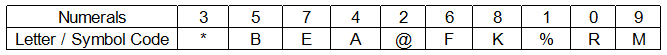## IBPS RRB Reasoning Test 13

Instructions

In each of the questions below, a group of numerals is given followed by four groups of symbol/letter combinations numbered a:, b:, c: and d:. Numerals are to be coded as per the codes and conditions given below. You have to nd out which of the combinations a:, b:, c: and d: is correct and indicate your answer accordingly. If none of the four combinations represent the correct code, mark e: as your answer.Following conditions apply :
(i) If the first digit as well as the last digit is odd, both are to be coded as ‘X’.
(ii) If the first digit as well as the last digit is even, both are to be coded as ‘\$’.
(iii) If the last digit is ‘0’, it is to be coded as ‘#’.

Q 1

546839

Q 2

765082

Q 3

364819

Q 4

487692

Q 5

713540